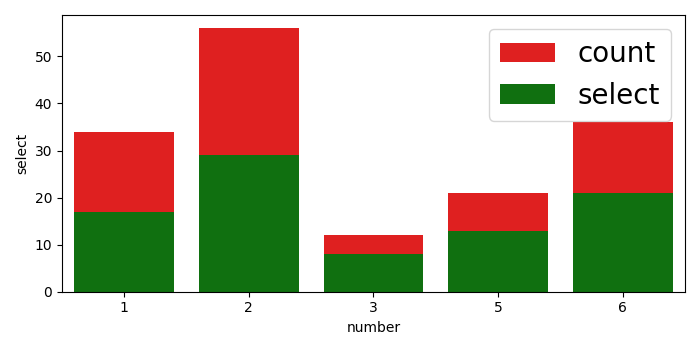# How to increase the font size of the legend in my Seaborn plot using Matplotlib?

To increase the font size of the legend in a Seaborn plot, we can use the fontsize variable and can use it in legend() method argument.

## Steps

• Create a data frame using Pandas. The keys are number, count, and select.

• Plot a bar in Seaborn using barplot() method.

• Initialize a variable fontsize to increase the fontsize of the legend.

• Use legend() method to place legend on the figure with fontsize in the argument.

• To display the figure, use show() method.

## Example

import pandas
import matplotlib.pylab as plt
import seaborn as sns
plt.rcParams["figure.figsize"] = [7.00, 3.50]
plt.rcParams["figure.autolayout"] = True
df = pandas.DataFrame(dict(
number=[2, 5, 1, 6, 3],
count=[56, 21, 34, 36, 12],
select=[29, 13, 17, 21, 8]
))
bar_plot1 = sns.barplot(x='number', y='count', data=df, label="count", color="red")
bar_plot2 = sns.barplot(x='number', y='select', data=df, label="select", color="green")
fontsize = 20
plt.legend(loc="upper right", frameon=True, fontsize=fontsize)
plt.show()

## Output